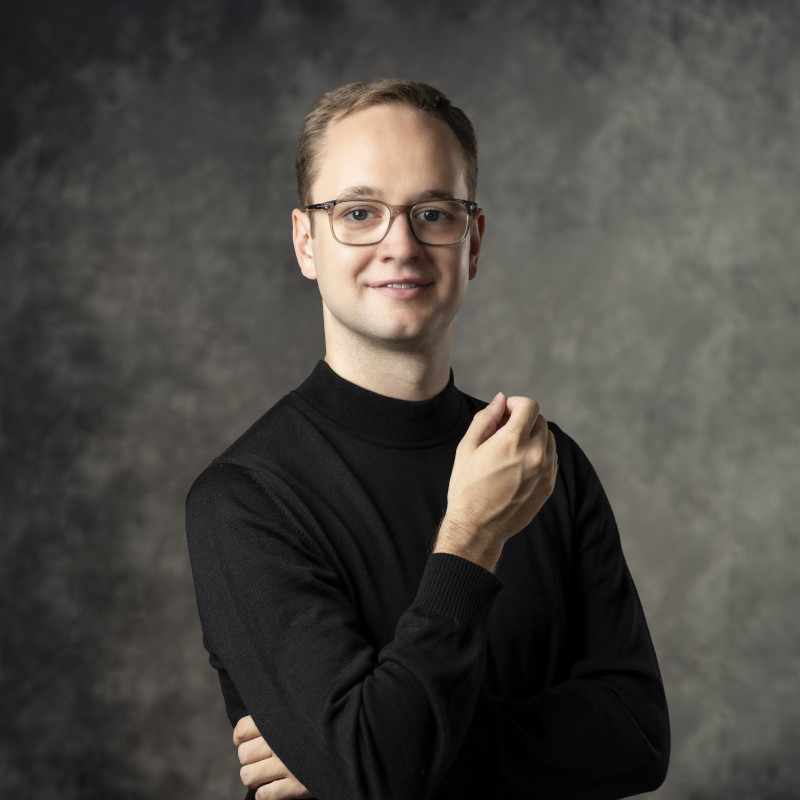# Florian KranholdI have moved to the Karlsruhe. My new homepage is here.
Address Karlsruhe Institute of Technology Englerstraße 2 76131 Karlsruhe Kollegiengebäude, 1.019 PGP Public Key (0xD2890F65)

I am a postdoctoral researcher at the Karlsruhe Institute of Technology in the working group of Manuel Krannich, specialising in the area of algebraic topology. Before that, I was a doctoral and a postdoctoral researcher at the Mathematical Institute of the University of Bonn, in the working group of Carl-Friedrich Bödigheimer.

My research interests lie in the area of algebraic topology: more specifically, I am interested in (coloured) topological operads andtheir algebras, configuration spaces and their homological stability, as well as diffeomorphism groups and mapping class groups, moduli spaces of surfaces and their (unstable) homology. More recently, I have been studying low-dimensional equivariant and parametrised cobordism cate­go­ries and their homotopy type.

Here is my CV and my ORCID record, and you can watch a Zoom talk I gave in the Purdue Topology Seminar in September 2021, as well as a Talk at a blackboard I gave at a Workshop in Co­pen­hagen in November 2021.

### Research

• #### Computations in the unstable homology of moduli spaces of Riemann surfaces

In this article we give a survey of homology computations for moduli spaces $$\smash{\mathfrak{M}_{\smash{g,1}}^m}$$ of Riemann surfaces with genus $$g\geqslant 0$$, one boundary curve, and $$m\geqslant 0$$ punctures. While rationally and stably this question has a satisfying answer by the Madsen–Weiss theorem, the unstable homology remains notoriously complicated. We discuss calculations with integral, mod-2, and rational coefficients. Furthermore, we determine, in most cases, explicit generators using homology operations

• #### Parametrised moduli spaces of surfaces as infinite loop spaces

We study the $$E_2$$-algebra $$\Lambda\mathfrak{M}_{*,1}=\coprod_{g\geqslant 0}\Lambda\mathfrak{M}_{g,1}$$ consisting of free loop spaces of moduli spaces of Riemann surfaces with one parametrised boundary component, and compute the homotopy type of the group completion $$\Omega B\Lambda\mathfrak{M}_{*,1}$$: it is the product of $$\Omega^\infty\mathbf{MTSO}(2)$$ with a certain free $$\Omega^\infty$$-space depending on the family of all boundary-irreducible mapping classes in all mapping class groups $$\Gamma_{g,n}$$ with $$g\geqslant 0$$ and $$n\geqslant 1$$.

• #### Configuration spaces of clusters as $$E_d$$-algebras

It is a classical result that configuration spaces of labelled particles in $$\mathbb{R}^d$$ are free algebras over the little $$d$$-cubes operad $$\mathscr{C}_d$$, and their $$d$$-fold bar construction is equivalent to the $$d$$-fold suspension of the labelling space. The aim of this paper is to study a variation of these spaces, namely the configuration space of labelled clusters of points in $$\mathbb{R}^d$$. This configuration space is again an $$E_d$$-algebra, but in general not a free one. We give geometric models for their iterated bar construction in two different ways: one uses an additional verticality constraint, and the other one uses a description of these clustered configuration spaces as cellular $$E_1$$-algebras. In the last section, we show a stable splitting result and present some applications.

• #### Vertical configuration spaces and their homology

We introduce ordered and unordered configuration spaces of ‘clusters’ of points in an Euclidean space $$\mathbb{R}^d$$, where points in each cluster have to satisfy a ‘verticality’ condition, depending on a decomposition $$d=p+q.$$ We compute the homology in the ordered case and prove homological stability in the unordered case.

### Theses

• #### Coloured topological operads and moduli spaces of surfaces with multiple boundary curves

This is my PhD thesis which was finished in January 2022 under the supervision of Carl-Friedrich Bödigheimer.

• #### Moduli spaces of Riemann surfaces and symmetric products: A combinatorial description of the Mumford–Miller–Morita classes

This is my master thesis which was finished in the summer term 2018 under the supervision of Carl-Friedrich Bödigheimer. Its main result is a Poincaré–Lefschetz correspondence between the Mumford–Miller–Morita classes in the cohomology of moduli spaces and certain subcomplexes of Bödigheimer’s simplicial model of parallel slit domains.

### Teaching

In the summer term 2022, I was a teaching assistant for the lectures Einführung in die Geometrie und Topologie by Koen van den Dungen and Topology 2 by Daniel Kasprowski.

In the summer term 2020, I co-organised a graduate seminar on Operads in Algebra and Topology together with Andrea Bianchi. Moreover, I have been tutor for the following topology lectures:

 SoSe 2021 Algebraic Topology 2 Carl-Friedrich Bödigheimer WiSe 2020/21 Algebraic Topology 1 Carl-Friedrich Bödigheimer SoSe 2020 Algebraic Topology 2 Christoph Winges WiSe 2019/20 Algebraic Topology 1 Wolfgang Lück SoSe 2019 Topology 2 Daniel Kasprowski WiSe 2018/19 Topology 1 Wolfgang Lück SoSe 2018 Einführung in die Geometrie und Topologie Wolfgang Lück